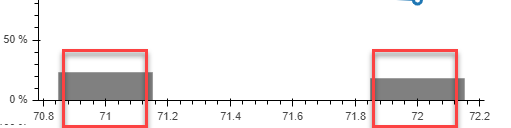# vbar x_range display

Hi guys,

I plotted the figure with some vbars. The x-axis is based on a list, let us say: [71, 72].

If I like to display just these two numbers, how could I do?

Currently, my result is as the screenshot shown below.

welcome any hints.

Thanks a lot.This should help:
import numpy as np
from bokeh.plotting import figure, show

x = np.linspace(0, 10, 1000)
plot = figure()
plot.line(x = x, y = np.log(x) * np.sin(x))
plot.xaxis.ticker.desired_num_ticks = 2

show(plot)

``

···

On Tuesday, January 8, 2019 at 12:50:28 AM UTC+1, peng wang wrote:

Hi guys,

I plotted the figure with some vbars. The x-axis is based on a list, let us say: [71, 72].

If I like to display just these two numbers, how could I do?

Currently, my result is as the screenshot shown below.

welcome any hints.

Thanks a lot.